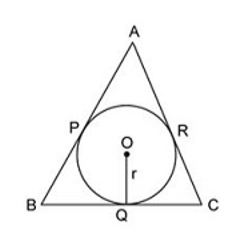# SSC CHSL 7 March 2018 Afternoon Shift

Instructions

For the following questions answer them individually

Question 1

The length of a string is 8 feet and 9 inches, which is divided into 3 equal parts. What is the length of each part in inches?

Question 2

Determine the largest 4 digit number which is a perfect square.

Question 3

Shaan got a total of Rs. 912 in the denomination of equal numbers of Rs. 1, Rs. 5 and Rs. 10 coins. How many coins do Shaan posses?

Question 4

Two whole numbers are such that the square of first number exceeds the second by 112 and the ratio of the numbers is 4:3. What is the value of smaller number?

Question 5

Choose the CORRECT option about the two tangents drawn to the circle from the external point?
1. The tangents subtend equal angles at the centre of the circle.
2. The tangents drawn from the external point to a circle are equal in length.
3. The tangents are equally inclined to the segment which joins the centre to that point.

Question 6

Consider the figure shown below which consists of the triangle ABC which touches the circle with centre at O. Which of the following options is CORRECT ?Question 7

If price of oranges is increased by 30%, then a person can buy 12 oranges less for Rs 208. What is the original price (in Rs) of an orange?

Question 8

Of three positive numbers, the ratio of first and second is 5: 2, ratio of second and third is 5: 4. The product of first and third is 1800. What is the sum of the three numbers?

Question 9

The ratio of milk and water in three samples is 1: 3, 3: 5 and 11: 5. A mixture containing equal quantities of all three samples is made. What will be the ratio of milk and water in the new mixture?

Question 10

Average of 31 numbers is 19. If the average of first 8 numbers is 24, then what is the average of the remaining numbers?

OR